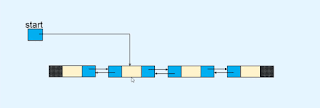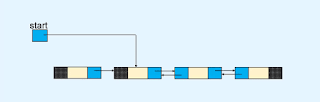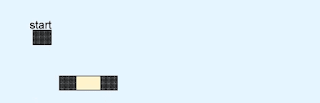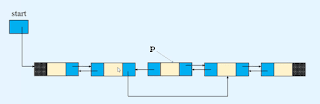In this tutorial, we are going to learn how to perform deletion operations in the doubly linked list.

after completing this tutorial you are able to learn how to
1. Delete the first node.
2. Delete the only node.
3. Delete a node in between the nodes.
4. Delete the last node.

### Deletion of the first node from the doubly linked list.

as you see we have a doubly-linked list that has four nodes. so after deleting the first node second node become the first node.so first we store the second node's reference to the start variable.and then we set the second node's previous link part to Null or None. because the previous link part of the first node in the doubly linked list is always None or Null.so after performing these steps, the first node is deleted.### Delete the only node from the doubly linked list.

if a linked list has only one node then to delete this node we set the start variable to the None.so now our list becomes an empty list.

### Delete a node in between the nodes.

to delete a node between two nodes first we need to find out the reference to the node that we want to delete. as you see to delete the third node first we found a reference p to that node.and then we store the reference of the node that comes after node p into the next link part of the node that comes before node p.and then we store the reference of the node that comes before node p into the previous link part of the node that comes after node p.now after performing these steps now, the third node has deleted from the doubly linked list.### Delete the last node from the doubly linked list.

to delete the last node of the list first we need to find out the reference of the last node.and then we set the next link part of the node to Null or None that come before node p.so after performing these steps now the last node of the list is deleted.#### Python code to delete the node in the Doubly linked list

```class Node(object):

def __init__(self, value):
self.info = value
self.prev = None        self.next = None

def __init__(self):
self.start = None
def display_list(self):
if self.start is None:
print("LIst is empty")
return
print("List is : ")
p = self.start
while p is not None:
print(p.info, " ", end='')
p = p.next
print()

def insert_in_beginning(self, data):
temp = None(data)
temp.next = self.start
self.start.prev = temp
self.start = temp

def insert_in_empty_list(self, data):
temp = Node(data)
self.start = temp

def insert_at_end(self, data):
temp = Node(data)
p = self.start
while p.next is not None:
p = p.next
p.next = temp
temp.prev = p

def create_list(self):
n = int(input("Enter the number of nodes : "))
if n == 0:
return        data = int(input("Enter the first element to be inserted : "))
self.insert_in_empty_list(data)

for i in range(n - 1):
data = int(input("Enter the next element to be inserted : "))
self.insert_at_end(data)

def insert_after(self, data, x):
temp = Node(data)
p = self.start
while p is not None:
if p.info == x:
break            p = p.next

if p is None:
print(x, " not present in the list")
else:
temp.prev = p
temp.next = p.next
if p.next is not None:
p.next.prev = temp
p.next = temp

def insert_before(self, data, x):
if self.start is None:
print("List is empty")
return
if self.start.info == x:
temp = Node(data)
temp.next = self.start
self.start.prev = temp
self.start = temp
return
p = self.start
while p is not None:
if p.info == x:
break            p = p.next

if p is None:
print(x, " not present in the list")
else:
temp = Node(data)
temp.prev = p.prev
temp.next = p
p.prev.next = temp
p.prev = temp

def delete_first_node(self):
if self.start is None:
return        if self.start.next is None:
self.start = None            return        self.start = self.start.next
self.start.prev = None
def delete_last_node(self):
if self.start is None:
return        if self.start.next is None:
self.start = None            return
p = self.start
while p.next != None:
p = p.next
p.prev.next = None
def delete_node(self, x):
if self.start is None:
return        if self.start.next is None:
if self.start.info == x:
self.start = None            else:
return
if self.start.info == x:
self.start = self.start.next
self.start.prev = None            return
p = self.start.next
while p.next is not None:
if p.info == x:
break
p = p.next

if p.next is not None:
p.prev.next = p.next
p.next.prev = p.prev
else:
if p.info == x:
p.prev.next
else:

def reverse_list(self):
if self.start is None:
return
p1 = self.start
p2 = p1.next
p1.next = None        p1.prev = p2
while p2 is not None:
p2.prev = p2.next
p2.next = p1
p1 = p2
p2 = p2.prev
self.start = p1

#################################################################3

list.create_list()

while True:
print("1. Display list")
print("2. Insert in empty list")
print("3. Insert a node in the beginning of the list")
print("4. Insert a node at the end of the list")
print("5. Insert a node after a specified node")
print("6. Insert a node before a specified node")
print("7. Delete first node")
print("8. Delete last node")
print("9. Delete any node")
print("10. Reverse the list")
print("11. Quit")

option = int(input("Enter your choice : "))

if option == 1:
list.display_list()
elif option == 2:
data = int(input("Enter the element to be inserted : "))
list.insert_in_empty_list(data)
elif option == 3:
data = int(input("Enter the element to be inserted : "))
list.insert_in_beginning(data)
elif option == 4:
data = int(input("Enter the element to be inserted : "))
list.insert_at_end(data)
elif option == 5:
data = int(input("Enter the element to be inserted : "))
x = int(input("Enter the element after which to insert : "))
list.insert_after(data, x)
elif option == 6:
data = int(input("Enter the element to be inserted : "))
x = int(input("Enter the element before which to inserted : "))
list.insert_before(data, x)
elif option == 7:
list.delete_first_node()
elif option == 8:
list.delete_last_node()
elif option == 9:
data = int(input("Enter the element to be deleted : "))
list.delete_node(data)
elif option == 10:
list.reverse_list()
elif option == 11:
break    else:
print("Wrong option")
print()```